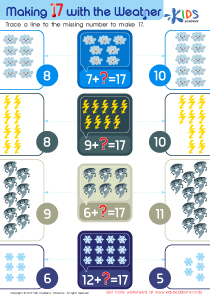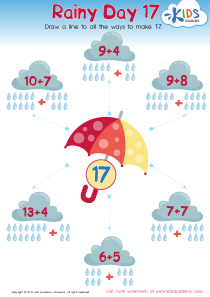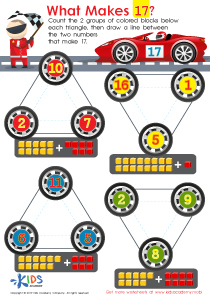# Math Lesson - Making 17, Kindergarten

• ### Activity 1 / Making 17 with the Weather WorksheetBefore beginning this addition exercise, show your kids the pictures in this worksheet. Can they identify the different kind of weathers shown in the tracing sheet? The aim of this exercise is to help your children get better at adding simple numbers. There are 4 different equations in the printout. Help your kids figure out the missing numbers that would make 17, and then trace a line to the missing number to make 17.

• ### Activity 2 / Rainy Day 17 WorksheetHelp your children with the simple equations shown. There are 6 different equations in the printout. Each cloud shows the addition of two numbers, and some of these numbers make 17. Help your kids figure out the total of both numbers when added, and then trace a line to the umbrella in the middle to show all the ways to make 17.  At the end of the exercise, they would be better at adding simple equations.

• ### Activity 3 / What Makes 17 WorksheetAddition and subtraction might prove a little difficult for your students, but with enough practice nothing is too difficult for your little kindergartners. Use this worksheet to show them how easy addition can really be. When we add different sets of numbers, sometimes we can arrive at the same total. Count the 2 groups of colored blocks below each triangle and then draw a line between the two numbers that make 17.

?